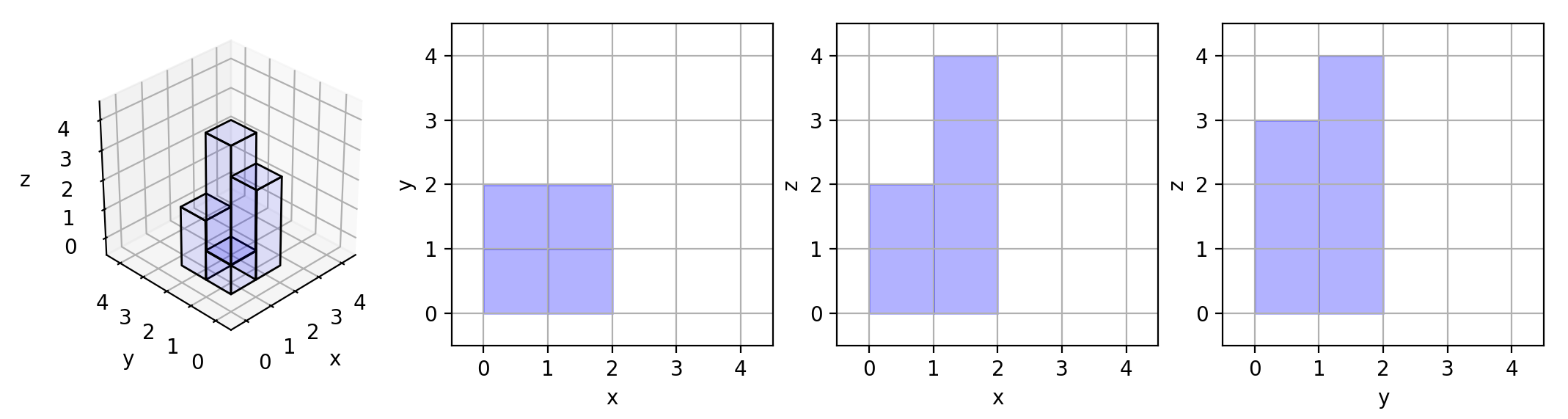Comment on page

# 883.Projection Area of 3D Shapes

883.Projection Area of 3D Shapes1 <= grid.length = grid.length <= 50
0 <= grid[i][j] <= 50

class Solution {
public:
int projectionArea(vector<vector<int>>& grid) {
int r=grid.size();
if(!r) return 0;
int c=grid.size();
int sum=0;
for(auto x:grid)
sum+= *max_element(x.begin(),x.end());
for(int i=0;i<c;i++)
{
int mc=0;
for(int j=0;j<r;j++)
{
mc=max(mc,grid[j][i]);
if(grid[j][i]) sum++;
}
sum +=mc;
}
return sum;
}
};